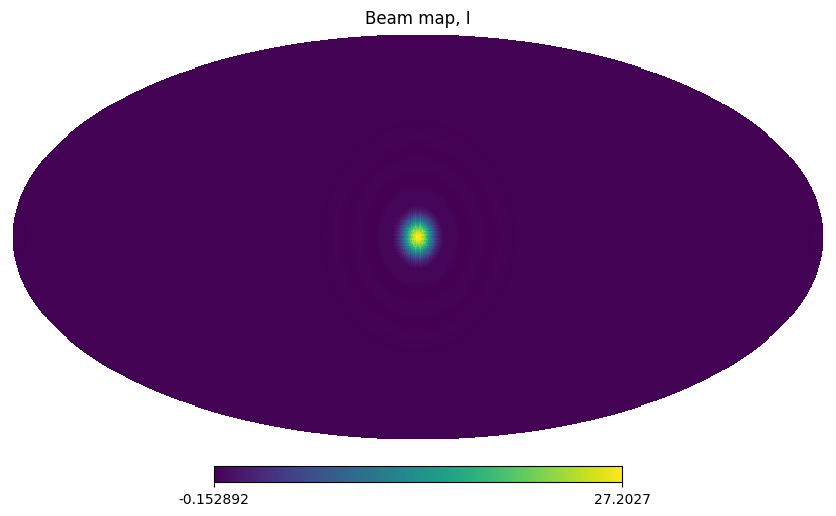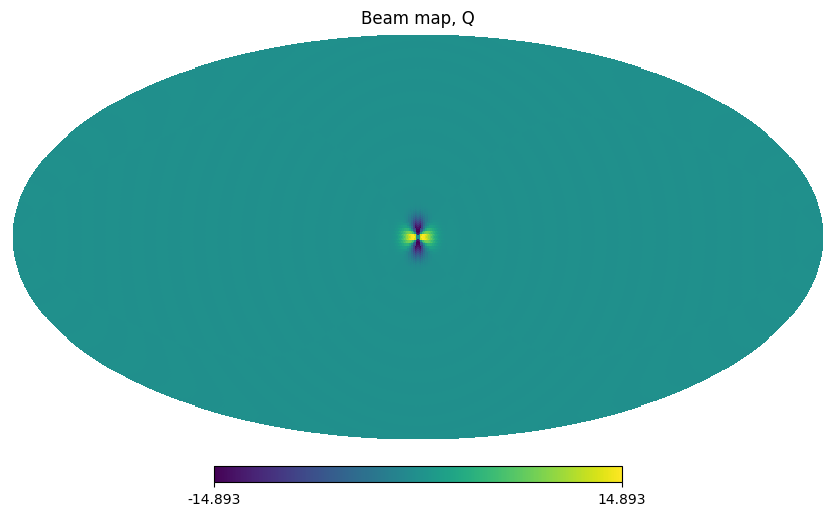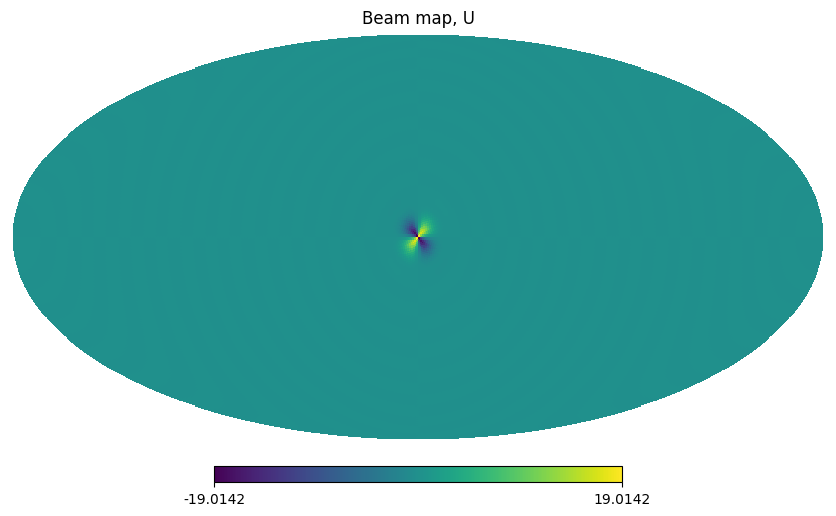# Example of generating a Gaussian beam in spherical harmonics space¶

Generate $$b_{lm}$$ representation of a Gaussian beam

:

import healpy as hp
import numpy as np
import matplotlib.pyplot as plt
from astropy import units as u


Configure parameters of the transformation

:

lmax = 32
pol = True
nside = 64
beam_width = 10 * u.degree


## Generate the $$b_{lm}$$¶

The shape is $$(1, \ell_{max})$$ for temperature only, $$(3, \ell_{max})$$ for a polarized beam.

:

blm=hp.blm_gauss(beam_width.to_value(u.radian), lmax=lmax, pol=pol)

:

blm.shape

:

(3, 96)


## Plot¶

Plot the beam, rotate inside mollview for better visualization

:

m = hp.alm2map(blm, lmax=lmax, mmax=2 if pol else 0, nside=nside, pol=pol)

:

for each_m, label in zip(m, "IQU"):
hp.mollview(each_m, rot=[0, 90], title=f"Beam map, {label}")[ ]: